# 2D shapes

Here you will learn about 2D shapes, including the attributes of various 2D shapes and symmetry.

Students first learn about 2D shapes in kindergarten and first grade with their work in geometry, identifying and classifying shapes by their properties.

They expand their knowledge of 2D shapes as they progress through elementary and middle school.

## What are 2D shapes?

2D shapes are flat shapes that can be drawn on a flat surface (or plane).

They have two dimensions, length and width, and have no thickness.

2D shapes can be grouped by the dimensions they have.

Here are some familiar 2D shapes.

### Polygons

Polygons are 2D shapes made from straight lines that meet at points called vertices.

There are two different types of polygons.

Regular polygonsIrregular polygons

Regular polygons have equal side
lengths and equal interior angles.

Interior angles are the angles
inside the polygon.

Equilateral Triangle

Square

Regular Hexagon

Irregular polygons do not have all equal
sides and do not have all equal angles.

Interior angles are the angles
inside the polygon.

Right Triangle

Rectangle

Irregular Hexagon

Step-by-step guide: Polygons

Step-by-step guide: Regular polygons

Step-by-step guide: Irregular polygons

### Symmetry

Symmetry is when an object can be divided into equal halves, where the two halves are mirror images of each other.

Line symmetry

Line symmetry is when an object can be divided in half with the two halves being
reflections of each other.

Both regular polygons and irregular polygons can have lines symmetry.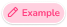Square

A square has 4 lines of symmetry.Equilateral Triangle

An equilateral triangle has 3 lines of symmetry.

The lines of symmetry of a regular polygon are equal to the number of sides.Trapezoid

This trapezoid is not a regular polygon and has only 1 line of symmetry.

Step-by-step guide: Line symmetry

Step-by-step guide: Symmetry

### Properties of 2D Shapes

Let’s look at the properties of some of the most common 2D Shapes.

Name of polygonProperties of polygon

Equilateral triangle

3 vertices
3 equal sides
3 equal angles
● Each interior angle is 60^{\circ}
3 lines of symmetry
3 times of rotational symmetry
within one full turn

Square

4 vertices
4 equal sides
4 equal angles
● Each interior angle is 90^{\circ}
4 lines of symmetry
4 times of rotational symmetry
within one full turn

Regular pentagon

5 vertices
5 equal sides
5 equal angles
● Each interior angle is 108^{\circ}
5 lines of symmetry
5 times of rotational symmetry
within one full turn

Regular hexagon

6 vertices
6 equal sides
6 equal angles
● Each interior angle is 120^{\circ}
6 lines of symmetry
6 times of rotational symmetry
within one turn

Regular octagon

8 vertices
8 equal sides
8 equal angles
● Each interior angle is 135^{\circ}
8 lines of symmetry
8 times of rotational symmetry
within one turn

Step-by-step guide: Pentagon

Step-by-step guide: Hexagon

Step-by-step guide: How to draw a hexagon

Step-by-step guide: Octagon

### What are 2D shapes?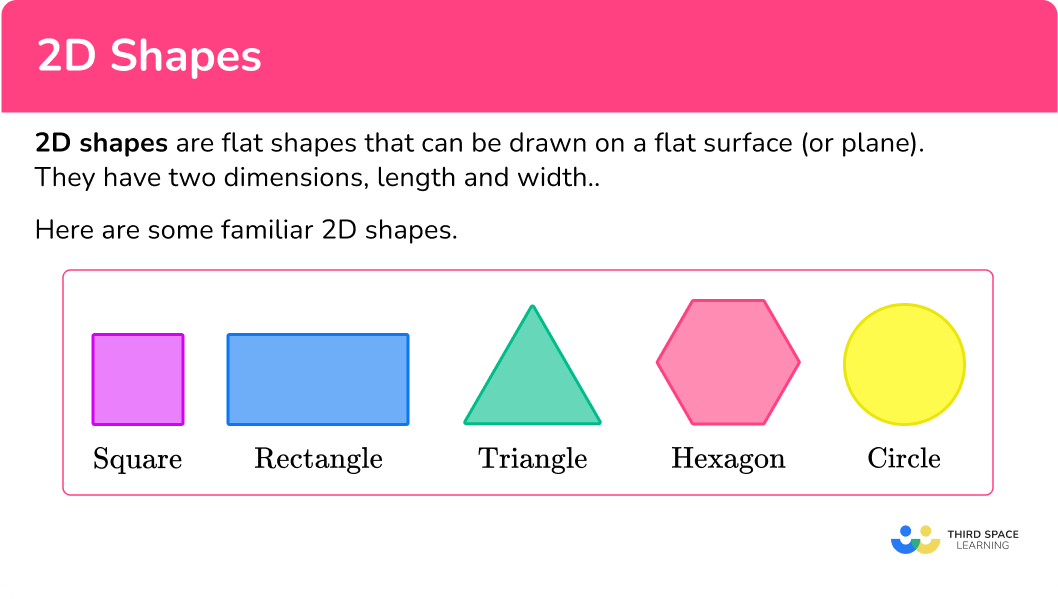## Common Core State Standards

How does this relate to kindergarten – 5 th grade math?

• Kindergarten: Geometry ( K.G.A.3)
Identify shapes as two-dimensional (lying in a plane, “flat”) or three-dimensional (“solid”).

Compose two-dimensional shapes (rectangles, squares, trapezoids, triangles, half circles, and quarter-circles) or three-dimensional shapes (cubes, right rectangular prisms, right circular cones, and right circular cylinders) to create a composite shape and compose new shapes from the composite shape.

Recognize and draw shapes having specified attributes, such as a given number of angles or a given number of equal faces. Identify triangles, quadrilaterals, pentagons, hexagons, and cubes.

Recognize a line of symmetry for a two-dimensional figure as a line across the figure such that the figure can be folded along the line into matching parts. Identify line-symmetric figures and draw lines of symmetry.

Classify two-dimensional figures in a hierarchy based on properties.

## How to use 2D Shapes

There are a lot of ways to use 2D shapes. For more specific step-by-step guides, check out the individual pages linked in the “What are 2D shapes?” section above or read through the examples below.

In order to identify a 2D shape, a polygon, or a regular polygon.

1. Recall the definition.
2. Explain how the 2D shape fits the definition.

## 2D shapes examples

### Example 1: identifying polygons

What type of 2D shape is a stop sign?

1. Recall the definition.

A stop sign is a polygon with 8 sides.

2Explain how the 2D shape fits the definition.

Polygons that have 8 sides are called octagons. So, a stop sign is an octagon.

### Example 2: identifying a polygon

Is a circle a polygon?

A polygon is a 2D shape made up of straight lines that meet at points called vertices.

A circle is a 2D shape, but it is not made up of straight lines, so it is not a polygon.

### Example 3: identifying polygons

Is a pentagon a polygon?

A polygon is a 2D shape made up of straight lines.

A pentagon is a 2D shape made up of straight lines, so it is a polygon.

### Example 4: regular / irregular polygons

Is the parallelogram a regular polygon?

Regular polygons have equal side lengths and equal interior angles.

The parallelogram does not have equal side lengths and does not have equal interior angles, so it is not a regular polygon.

### Example 5: symmetry

How many lines of symmetry does this rectangle have?

Line symmetry is when an object can be divided in half, with the two halves being mirror images or reflections of each other.

In this case, the rectangle can be divided down the middle vertically and across the middle horizontally.

So there are 2 lines of symmetry.

### Example 6: symmetry

Name the shape.

Octagons are polygons with 8 sides.

Irregular polygons are polygons that do not have equal sides and equal angles.

The shape is an octagon because it has eight sides and eight angles. The sides and the angles are not equal, so it is also an irregular polygon. The shape is an irregular octagon.

### Teaching tips for 2D shapes

• Instead of relying on worksheets, use manipulatives and real world objects in class so that students can see and understand the different shapes.

• Link art projects to 2D shapes and symmetry so that students can apply the math to creative projects.

• Provide students with cut-outs of the actual shapes so that they can measure the side lengths and angle measurements to develop their understanding of regular shapes versus irregular shapes.

• Include in lesson plans math centers where your learners can rotate through various 2D shape activities that are comprised of hands-on activities with pattern blocks, shape flashcards, and digital activities with math games so that students can learn shapes and their attributes.

• Reinforce essential vocabulary as it pertains to the geometrical shapes so students can recall the shapes names and see how they are used in real life.

### Easy mistakes to make

• Confusing the different shapes
For example, thinking that a pentagon is a hexagon or vice versa.

• Thinking that a rectangle is a regular polygon
Squares are the only regular quadrilaterals. Rectangles have the same interior angles, but they do not have the same side lengths.

• Thinking that figures can’t have more than one line of symmetry
2D shapes can have more than one line of symmetry or no lines of symmetry.

• Rotational symmetry/lines of symmetry
Lines of symmetry are often confused with rotational symmetry. A line of symmetry on a two-dimensional shape divides the shape equally into two symmetrical pieces. Rotational symmetry is the number of times a shape fits into itself when rotated around its center.

• Thinking that an irregular hexagon is not a hexagon
If students have too much exposure to regular hexagons and not enough of irregular hexagons, they may believe that a regular hexagon is the only type of hexagon. Be sure to vary their exposure to hexagonal shapes and give them opportunities to work with and draw hexagons that are not regular. This applies to all other types of polygons as well.

### Practice 2D shapes questions

1. Which figure below represents a regular polygon?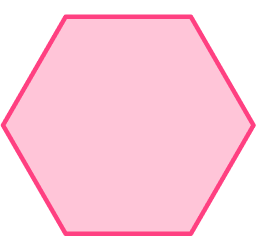Regular polygons have equal side lengths and equal interior angles.

The only figure that has equal side lengths and equal interior angles is the regular hexagon.

2. Which polygon has 5 vertices?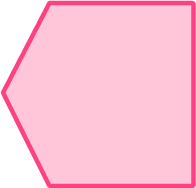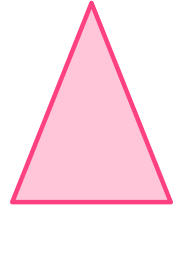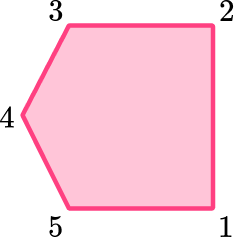The pentagon has five vertices.

The vertices are the corners of the shape.

3. Which 2D shape is an irregular hexagon?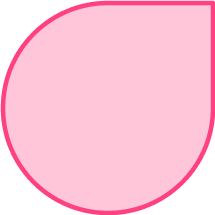An irregular hexagon is a 6 sided shape that does not have equal side lengths or equal interior angles.

This polygon was the only one with 6 sides.4. Which shape has 4 lines of symmetry?A square is a regular polygon with four equal sides and four equal interior angles.

So it will have four lines of symmetry.

A rectangle will only have two lines of symmetry, a kite and a parallelogram have no lines of symmetry.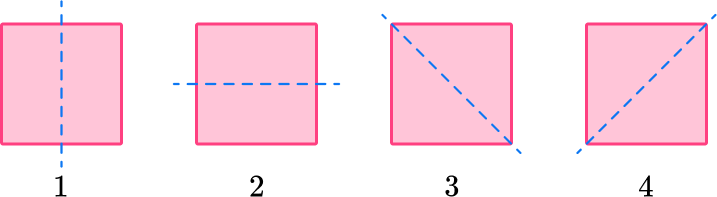5. How many lines of symmetry does this 2D figure have?38186By definition, line symmetry is when an object can be divided in half, with the two halves being mirror images or reflections of each other.

In this case, the snowflake has six lines of symmetry.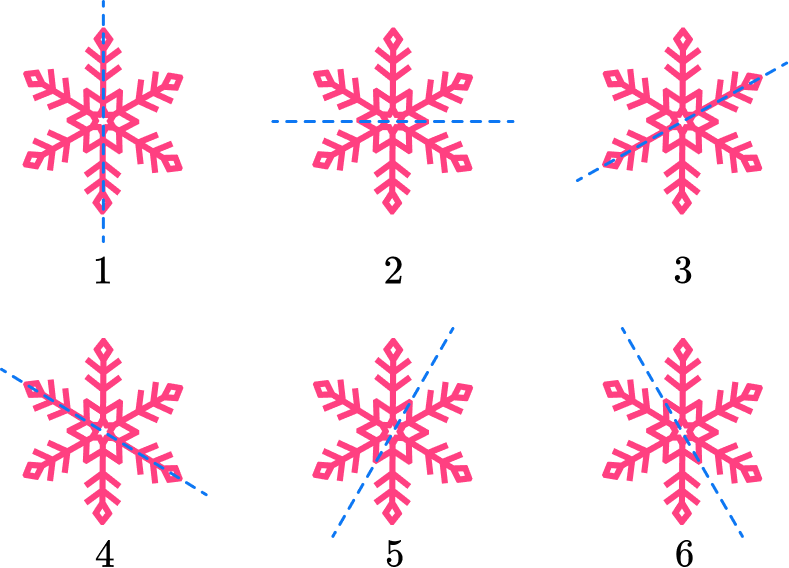6. Which of the following shapes has only two lines of symmetry?No lines of symmetry.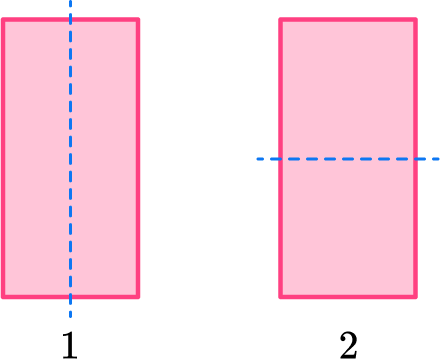Two lines of symmetryNo lines of symmetry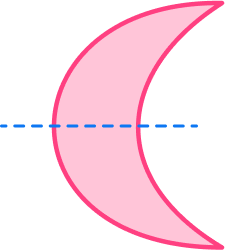One line of symmetry

## 2D shapes FAQs

Are geometric figures that are two dimensional with curved line edges polygons?

By definition, polygons are enclosed 2D shapes made up of straight lines that meet at points. If a shape has a curved line edge, it will not be a polygon.

How do you find the area and perimeter of polygons?

Perimeter is the distance around the shapes, so you can just add up the side lengths. The area is the space within the shapes. There are various formulas you will learn to find the areas of shapes such as triangles, squares, rectangles, and parallelograms.

How do you find the sum of the interior angle measurements of all regular polygons?

The sum of the interior angles of a polygon is always the number of sides minus 2 times 180 . You will learn this as you move into high school.

What is a cuboid?

A cuboid is another name for a cube, which are 3D shapes where each face is a square.

What is a rhombus?

A rhombus is a parallelogram, so opposite sides are equal and opposite sides are parallel, and in addition to that, all four sides are equal.

## Still stuck?

At Third Space Learning, we specialize in helping teachers and school leaders to provide personalized math support for more of their students through high-quality, online one-on-one math tutoring delivered by subject experts.

Each week, our tutors support thousands of students who are at risk of not meeting their grade-level expectations, and help accelerate their progress and boost their confidence.